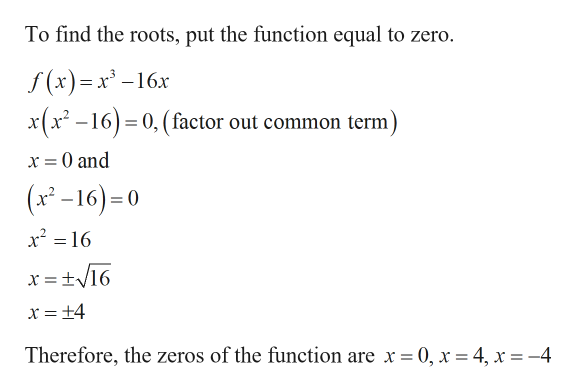# For each equation factor completely and find the horizontal intercepts (x-intercepts, zeros).f(x)=x3-4x-12x

Question
16 views

For each equation factor completely and find the horizontal intercepts (x-intercepts, zeros).

f(x)=x3-4x-12x

check_circle

Step 1

Given:

Step 2

Calculation:

Step 3

Zeros:

...help_outlineImage TranscriptioncloseTo find the roots, put the function equal to zero. f(x)=x° -16x x(x2-160, (factor out common term) x 0 and (x-16) x2 16 Therefore, the zeros of the function are x = 0, x = 4, x =-4 fullscreen

### Want to see the full answer?

See Solution

#### Want to see this answer and more?

Solutions are written by subject experts who are available 24/7. Questions are typically answered within 1 hour.*

See Solution
*Response times may vary by subject and question.
Tagged in

### Other